# Cluster Analysis Cluster Analysis p What is Cluster

• Slides: 26Cluster Analysis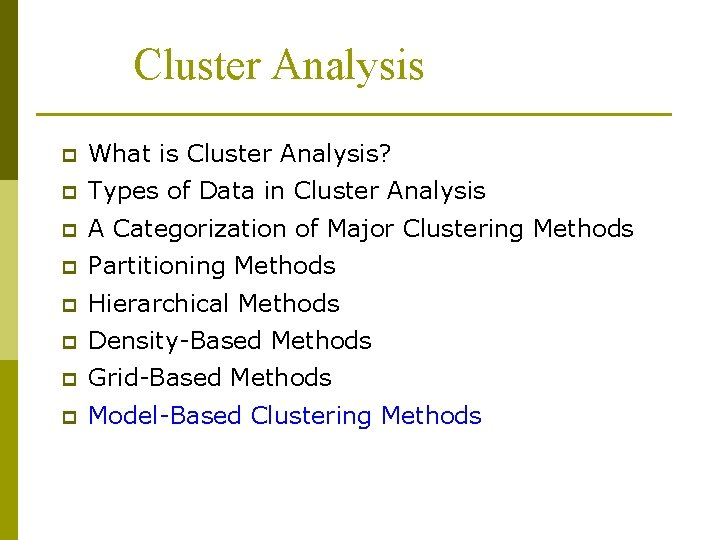Cluster Analysis p What is Cluster Analysis? p Types of Data in Cluster Analysis p A Categorization of Major Clustering Methods p Partitioning Methods p Hierarchical Methods p Density-Based Methods p Grid-Based Methods p Model-Based Clustering MethodsModel based clustering Assume data generated from K probability distributions p Typically Gaussian distribution Soft or probabilistic version of K-means clustering p Need to find distribution parameters. p EM Algorithm pEM Algorithm p p Initialize K cluster centers Iterate between two steps n Expectation step: assign points to clusters n Maximation step: estimate model parametersExpectation step p Assuming d-dim Gaussian distributions: Given the models, estimate the probability of a given record to belong to cluster h at iteration j as follows: where: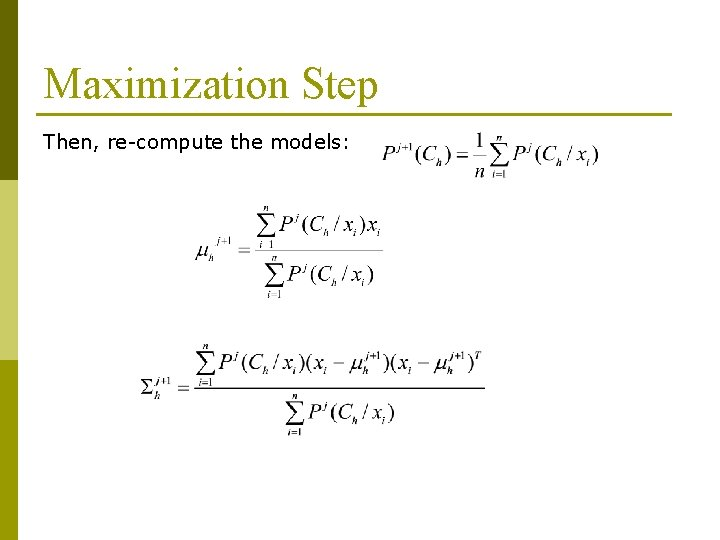Maximization Step Then, re-compute the models:Stoping criterion p Stop when: where: andCluster Analysis p What is Cluster Analysis? p Types of Data in Cluster Analysis p A Categorization of Major Clustering Methods p Partitioning Methods p Hierarchical Methods p Density-Based Methods p Grid-Based Methods p Model-Based Clustering Methods p Cluster ValidityCluster Validity p For supervised classification we have a variety of measures to evaluate how good our model is n Accuracy, precision, recall p For cluster analysis, the analogous question is how to evaluate the “goodness” of the resulting clusters? p But “clusters are in the eye of the beholder”! p Then why do we want to evaluate them? n n To To avoid finding patterns in noise compare clustering algorithms compare two sets of clusters compare two clustersClusters found in Random Data Random Points K-means DBSCAN Complete Link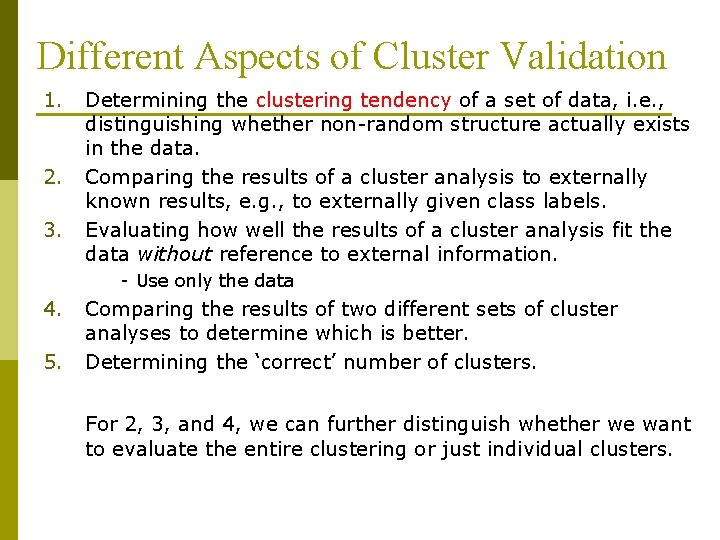Different Aspects of Cluster Validation 1. 2. 3. Determining the clustering tendency of a set of data, i. e. , distinguishing whether non-random structure actually exists in the data. Comparing the results of a cluster analysis to externally known results, e. g. , to externally given class labels. Evaluating how well the results of a cluster analysis fit the data without reference to external information. - Use only the data 4. 5. Comparing the results of two different sets of cluster analyses to determine which is better. Determining the ‘correct’ number of clusters. For 2, 3, and 4, we can further distinguish whether we want to evaluate the entire clustering or just individual clusters.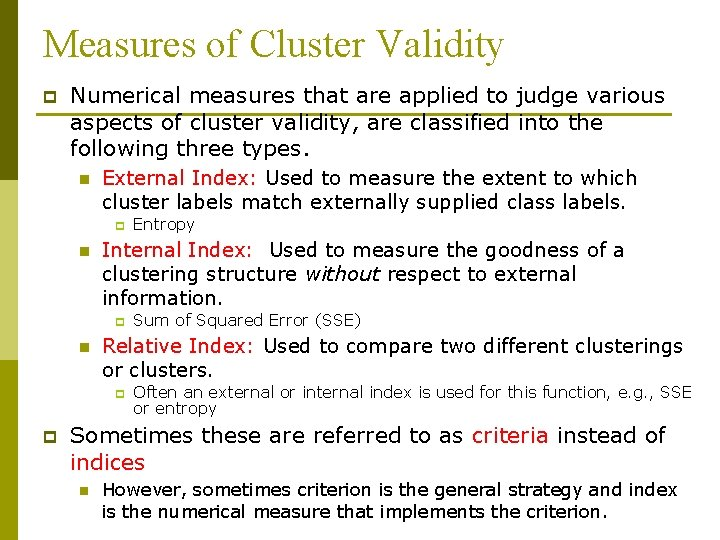Measures of Cluster Validity p Numerical measures that are applied to judge various aspects of cluster validity, are classified into the following three types. n External Index: Used to measure the extent to which cluster labels match externally supplied class labels. p n Internal Index: Used to measure the goodness of a clustering structure without respect to external information. p n Sum of Squared Error (SSE) Relative Index: Used to compare two different clusterings or clusters. p p Entropy Often an external or internal index is used for this function, e. g. , SSE or entropy Sometimes these are referred to as criteria instead of indices n However, sometimes criterion is the general strategy and index is the numerical measure that implements the criterion.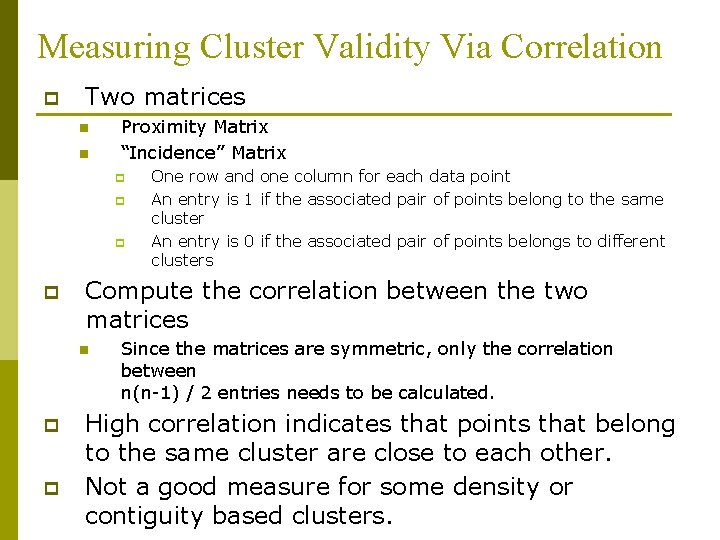Measuring Cluster Validity Via Correlation p Two matrices n n Proximity Matrix “Incidence” Matrix p p Compute the correlation between the two matrices n p p One row and one column for each data point An entry is 1 if the associated pair of points belong to the same cluster An entry is 0 if the associated pair of points belongs to different clusters Since the matrices are symmetric, only the correlation between n(n-1) / 2 entries needs to be calculated. High correlation indicates that points that belong to the same cluster are close to each other. Not a good measure for some density or contiguity based clusters.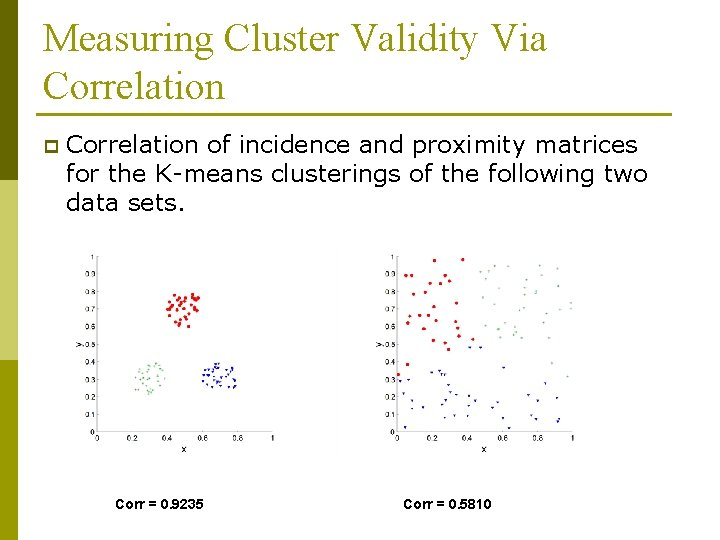Measuring Cluster Validity Via Correlation p Correlation of incidence and proximity matrices for the K-means clusterings of the following two data sets. Corr = 0. 9235 Corr = 0. 5810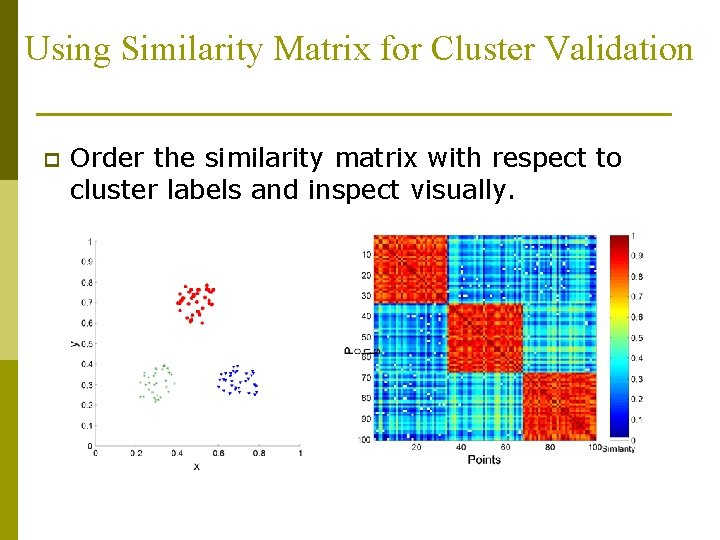Using Similarity Matrix for Cluster Validation p Order the similarity matrix with respect to cluster labels and inspect visually.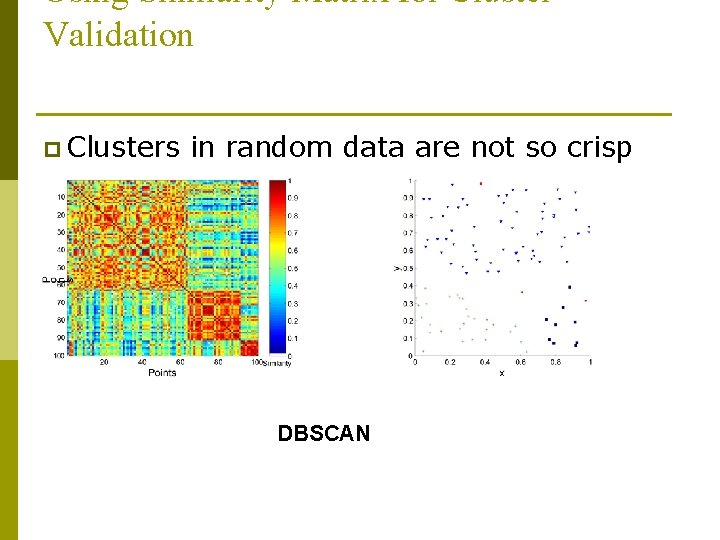Using Similarity Matrix for Cluster Validation p Clusters in random data are not so crisp DBSCAN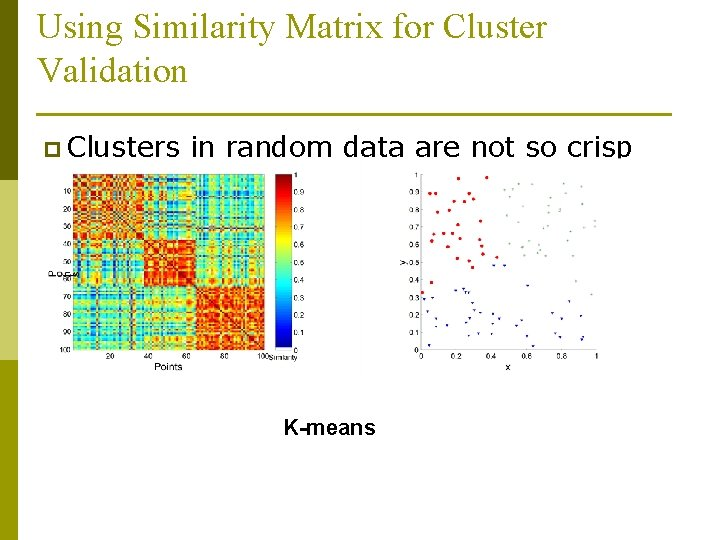Using Similarity Matrix for Cluster Validation p Clusters in random data are not so crisp K-means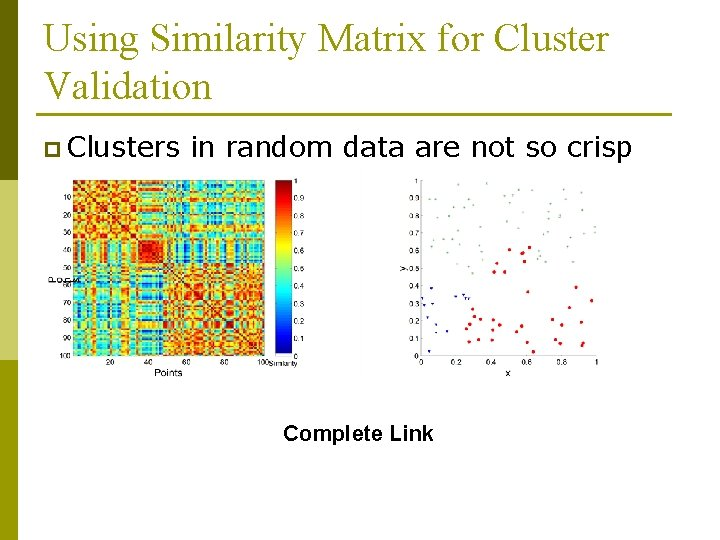Using Similarity Matrix for Cluster Validation p Clusters in random data are not so crisp Complete Link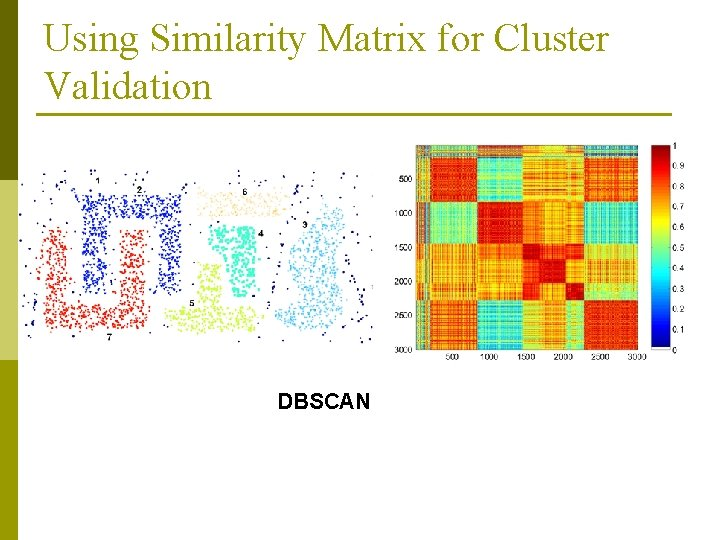Using Similarity Matrix for Cluster Validation DBSCAN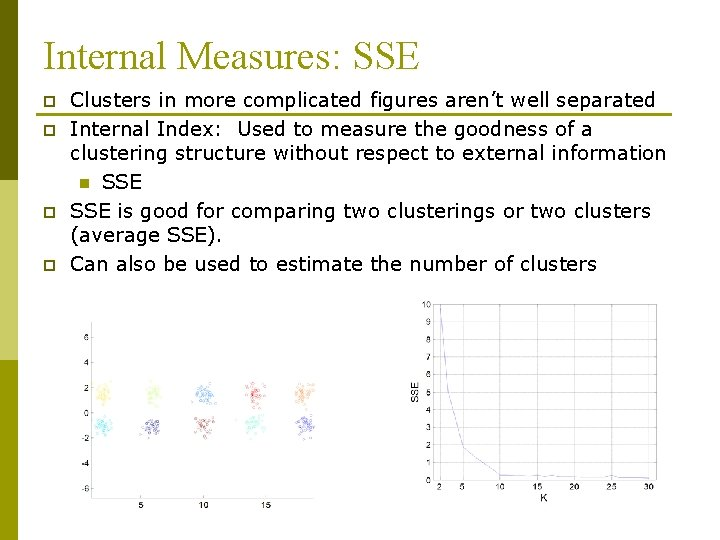Internal Measures: SSE p p Clusters in more complicated figures aren’t well separated Internal Index: Used to measure the goodness of a clustering structure without respect to external information n SSE is good for comparing two clusterings or two clusters (average SSE). Can also be used to estimate the number of clusters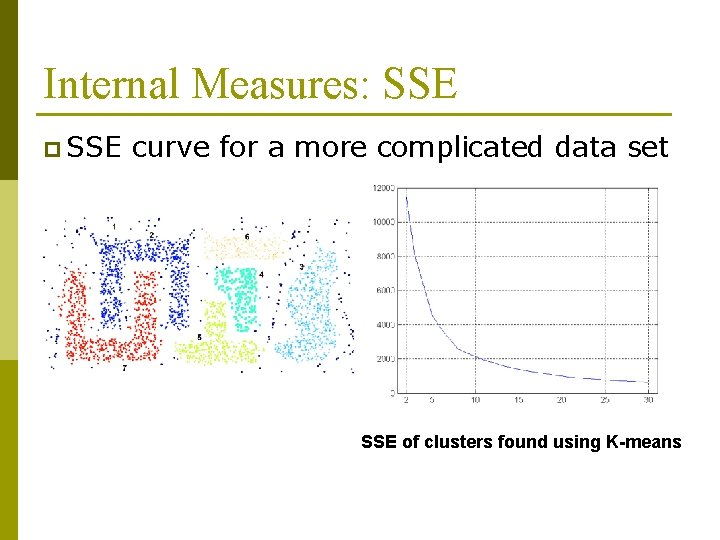Internal Measures: SSE p SSE curve for a more complicated data set SSE of clusters found using K-meansFramework for Cluster Validity p Need a framework to interpret any measure. n p For example, if our measure of evaluation has the value, 10, is that good, fair, or poor? Statistics provide a framework for cluster validity n n The more “atypical” a clustering result is, the more likely it represents valid structure in the data Can compare the values of an index that result from random data or clusterings to those of a clustering result. p n p If the value of the index is unlikely, then the cluster results are valid These approaches are more complicated and harder to understand. For comparing the results of two different sets of cluster analyses, a framework is less necessary. n However, there is the question of whether the difference between two index values is significant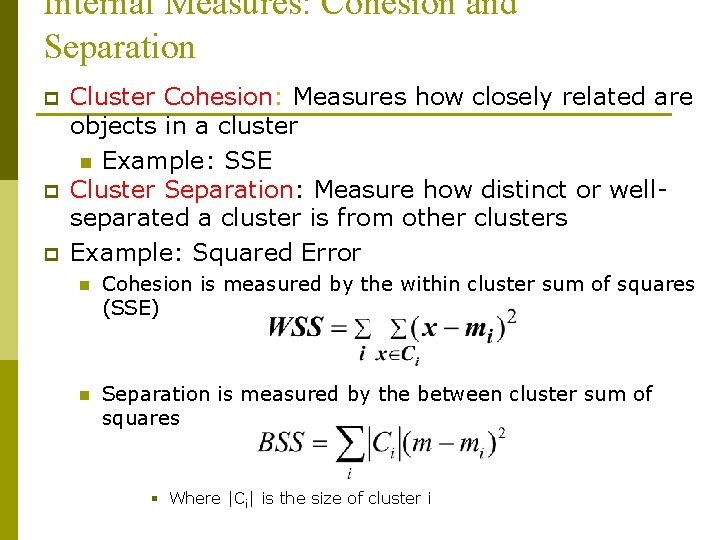Internal Measures: Cohesion and Separation p p p Cluster Cohesion: Measures how closely related are objects in a cluster n Example: SSE Cluster Separation: Measure how distinct or wellseparated a cluster is from other clusters Example: Squared Error n Cohesion is measured by the within cluster sum of squares (SSE) n Separation is measured by the between cluster sum of squares § Where |Ci| is the size of cluster i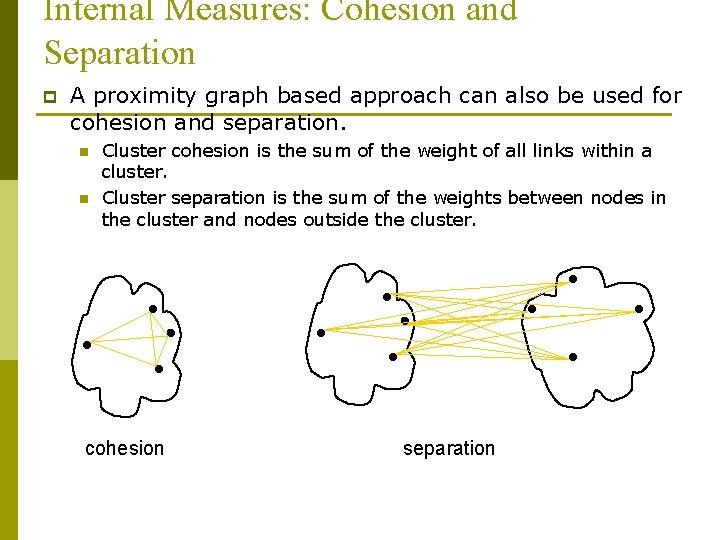Internal Measures: Cohesion and Separation p A proximity graph based approach can also be used for cohesion and separation. n n Cluster cohesion is the sum of the weight of all links within a cluster. Cluster separation is the sum of the weights between nodes in the cluster and nodes outside the cluster. cohesion separationInternal Measures: Silhouette Coefficient p p Silhouette Coefficient combine ideas of both cohesion and separation, but for individual points, as well as clusters and clusterings For an individual point, i n n n Calculate a = average distance of i to the points in its cluster Calculate b = min (average distance of i to points in another cluster) The silhouette coefficient for a point is then given by s = 1 – a/b n n p if a < b, (or s = b/a - 1 if a b, not the usual case) Typically between 0 and 1. The closer to 1 the better. Can calculate the Average Silhouette width for a clustering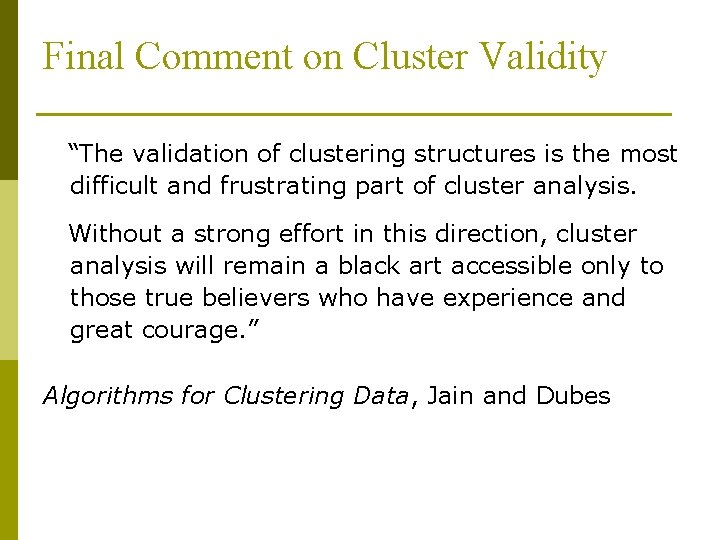Final Comment on Cluster Validity “The validation of clustering structures is the most difficult and frustrating part of cluster analysis. Without a strong effort in this direction, cluster analysis will remain a black art accessible only to those true believers who have experience and great courage. ” Algorithms for Clustering Data, Jain and Dubes